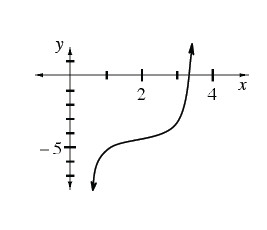### Home > CALC > Chapter 11 > Lesson 11.2.3 > Problem11-75

11-75.

Multiple Choice: $f(x)$, shown at right, is twice differentiable. Examine the graph and decide which statement below is true.

1. $f ( 2 ) < f ^ { \prime } ( 2 ) < f ^ { \prime \prime } ( 2 )$

1. $f ( 2 ) < f ^ { \prime \prime } ( 2 ) < f ^ { \prime } ( 2 )$

1. $f ^ { \prime } ( 2 ) < f ( 2 ) < f ^ { \prime \prime } ( 2 )$

1. $f ^ { \prime \prime } ( 2 ) < f ( 2 ) < f ^ { \prime } ( 2 )$

1. $f ^ { \prime \prime } ( 2 ) < f ^ { \prime } ( 2 ) < f ( 2 )$Approximate:
f(2)
f ′(2)
f ″(2)

f(2) < 0
f ′(2) > 0
f ″(2) ≈ 0